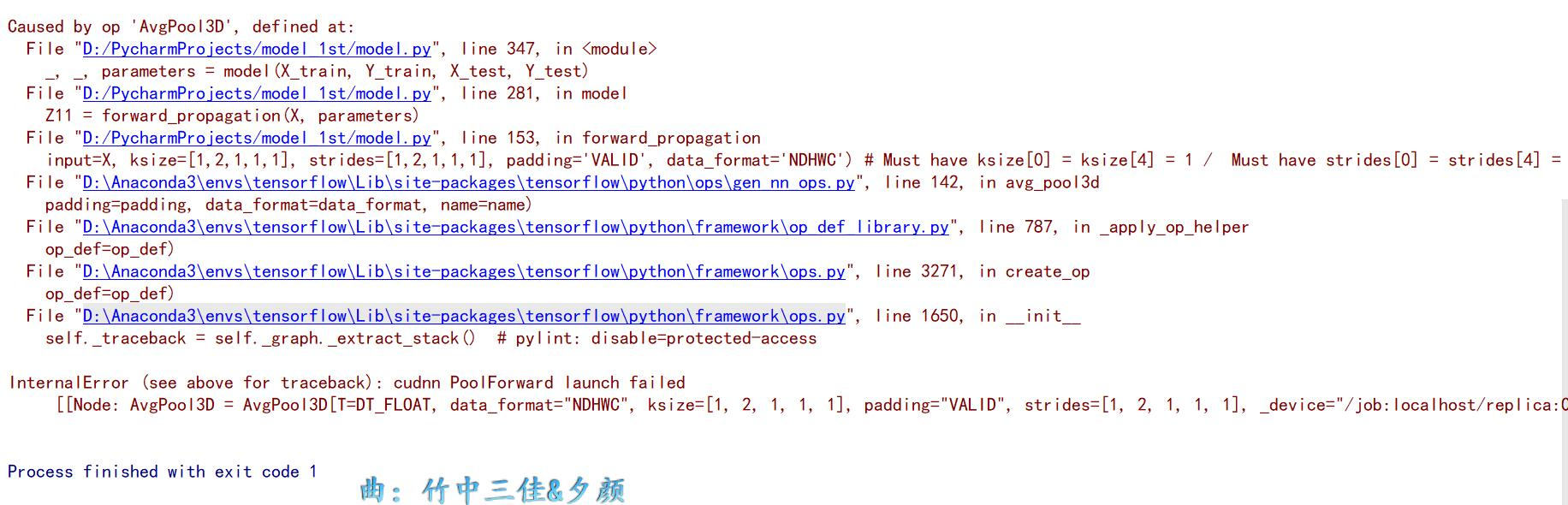gpu_options = tf.GPUOptions(per_process_gpu_memory_fraction=0.333)
sess = Session（config=tf.ConfigProto(gpu_options=gpu_options)）9 个月之前 回复

2个回答

https://github.com/tensorflow/tensorflow/issues/19567
pip install tf-nightly-gpu. This may help.TensorFlow实现卷积神经网络，出现类型转换异常
ERROR:tensorflow:Exception in QueueRunner: Cast string to int32 is not supported [[Node: Cast_1 = Cast[DstT=DT_INT32, SrcT=DT_STRING, _device="/job:localhost/replica:0/task:0/device:CPU:0"](Cast_1/x)]]
tensorflow中卷积神经网络输出层除了能做分类，还有哪些其他的输出层？

TensorFlow训练卷积神经网络中，输入数据必须是什么类型的？

TensorFlow卷积神经网络实现，图片与对应标签的拟合。一直显示卡在正在运行。
#python3代码 #TensorFlow卷积神经网络实现，图片与对应标签的拟合 #程序不报错，一直显示正在运行 #请问错在哪里？ #数据集 https://pan.baidu.com/s/1Z7_ULAW3LFrUk4WkHC0s3Q import os import numpy as np from PIL import Image import tensorflow as tf import matplotlib.pyplot as plt #-------------获取数据集，构造批数据：batch----------------------------------------- def get_files(file_dir): pictures = [] numbers = [] for file in os.listdir(file_dir): # 7000.jpg name = file.split(sep='.') pictures.append(file_dir+"\\"+file) numbers.append(name) print('There are %d pictures'%(len(pictures))) temp = np.array([pictures,numbers]) temp = temp.transpose() np.random.shuffle(temp) pictures_list = list(temp[:,0]) numbers_list = list(temp[:,1]) #numbers_list = [int(float(i)) for i in numbers_list] return pictures_list,numbers_list #返回两个list def get_batch(image,label,image_W,image_H,batch_size,capacity): # image = pictures label = numbers image = tf.cast(image,tf.string) label = tf.cast(label,tf.int32) #tf.cast()用来做类型转换 input_queue = tf.train.slice_input_producer([image,label]) #加入队列 label = input_queue image_contents = tf.read_file(input_queue) image = tf.image.decode_jpeg(image_contents,channels=3) #jpeg或者jpg格式都用decode_jpeg函数，其他格式可以去查看官方文档 image = tf.image.resize_image_with_crop_or_pad(image,image_W,image_H) #resize image = tf.image.per_image_standardization(image) #对resize后的图片进行标准化处理 image_batch,label_batch = tf.train.batch([image,label],batch_size = batch_size,num_threads=16,capacity = capacity) label_batch = tf.reshape(label_batch,[batch_size]) return image_batch,label_batch #获取两个batch，两个batch即为传入神经网络的数据 #-----------卷积神经网络节点，搭建卷积神经网络---------------------------------------------------- def conv_op(input_op,name,kh,kw,n_out,dh,dw): n_in = input_op.get_shape()[-1].value with tf.name_scope(name) as scope: kernel=tf.get_variable(scope+"w", shape=[kh,kw,n_in,n_out],dtype=tf.float32, initializer=tf.contrib.layers.xavier_initializer_conv2d()) conv=tf.nn.conv2d(input_op,kernel,(1,dh,dw,1),padding='SAME') bias_init_val=tf.constant(0.0,shape=[n_out],dtype=tf.float32) biases=tf.Variable(bias_init_val,trainable=True,name='b') z=tf.nn.bias_add(conv,biases) activation=tf.nn.relu(z,name=scope) return activation def fc_op(input_op,name,n_out): n_in=input_op.get_shape()[-1].value with tf.name_scope(name) as scope: kernel = tf.get_variable(scope+"w",shape=[n_in,n_out], dtype=tf.float32, initializer=tf.contrib.layers.xavier_initializer()) biases=tf.Variable(tf.constant(0.1, shape=[n_out],dtype=tf.float32),name='b') activation=tf.nn.relu_layer(input_op,kernel,biases,name=scope) return activation def fc_end_layer(inputs,in_size,out_size,activation_function=None): Weights=tf.Variable(tf.random_normal([in_size,out_size])) biases=tf.Variable(tf.zeros([1,out_size])+0.1) Wx_plus_b=tf.matmul(inputs,Weights)+biases if activation_function is None: outputs=Wx_plus_b else: outputs=activation_function(Wx_plus_b) return outputs def mpool_op(input_op,name,kh,kw,dh,dw): return tf.nn.max_pool(input_op,ksize=[1,kh,kw,1], strides=[1,dh,dw,1],padding='SAME',name=name) def inference_op(input_op,keep_prob): conv1_1=conv_op(input_op,name="conv1_1",kh=3,kw=3,n_out=64,dh=1, dw=1) conv1_2=conv_op(conv1_1,name="conv1_2",kh=3,kw=3,n_out=64,dh=1, dw=1) pool1=mpool_op(conv1_2,name="pool1",kh=2,kw=2,dw=2,dh=2) conv2_1=conv_op(pool1,name="conv2_1",kh=3,kw=3,n_out=128,dh=1, dw=1) conv2_2=conv_op(conv2_1,name="conv2_2",kh=3,kw=3,n_out=128,dh=1, dw=1) pool2=mpool_op(conv2_2,name="pool2",kh=2,kw=2,dh=2,dw=2) conv3_1=conv_op(pool2,name="conv3_1",kh=3,kw=3,n_out=256,dh=1, dw=1) conv3_2=conv_op(conv3_1,name="conv3_2",kh=3,kw=3,n_out=256,dh=1, dw=1) conv3_3=conv_op(conv3_2,name="conv3_3",kh=3,kw=3,n_out=256,dh=1, dw=1) pool3=mpool_op(conv3_3,name="pool3",kh=2,kw=2,dh=2,dw=2) conv4_1=conv_op(pool3,name="conv4_1",kh=3,kw=3,n_out=512,dh=1, dw=1) conv4_2=conv_op(conv4_1,name="conv4_2",kh=3,kw=3,n_out=512,dh=1, dw=1) conv4_3=conv_op(conv4_2,name="conv4_3",kh=3,kw=3,n_out=512,dh=1, dw=1) pool4=mpool_op(conv4_3,name="pool4",kh=2,kw=2,dh=2,dw=2) conv5_1=conv_op(pool4,name="conv5_1",kh=3,kw=3,n_out=512,dh=1, dw=1) conv5_2=conv_op(conv5_1,name="conv5_2",kh=3,kw=3,n_out=512,dh=1, dw=1) conv5_3=conv_op(conv5_2,name="conv5_3",kh=3,kw=3,n_out=512,dh=1, dw=1) pool5=mpool_op(conv5_3,name="pool5",kh=2,kw=2,dw=2,dh=2) shp=pool5.get_shape() flattened_shape=shp.value*shp.value*shp.value resh1=tf.reshape(pool5,[-1,flattened_shape],name="resh1") fc6=fc_op(resh1,name="fc6",n_out=4096) fc6_drop=tf.nn.dropout(fc6,keep_prob,name="fc6_drop") fc7=fc_op(fc6_drop,name="fc7",n_out=4096) fc7_drop=tf.nn.dropout(fc7,keep_prob,name="fc7_drop") fc8=fc_op(fc7_drop,name="fc8",n_out=1000) prediction=fc_end_layer(fc8,1000,1,activation_function=None) return prediction #---------构造损失节点：loss 优化节点：optimizer 训练节点:train--------------------------------------- x=tf.placeholder(tf.float32,[None,28,28,3]) y=tf.placeholder(tf.float32,[None,1]) prediction = inference_op(x,0.5) loss = tf.reduce_mean(tf.square(y - prediction)) optimizer = tf.train.GradientDescentOptimizer(0.5) train = optimizer.minimize(loss) #--------运行网络-------------------------------------------------- IMG_W = 28 IMG_H = 28 BATCH_SIZE = 4 CAPACITY = 24 MAX_STEP = 100 learning_rate = 0.0005 train_dir = 'D:\\VGGNet-16\\fatigue_cycle_picture' pictures_list,numbers_list = get_files(train_dir) image_batch,label_batch = get_batch(pictures_list,numbers_list,IMG_W,IMG_H,BATCH_SIZE,CAPACITY) #label_batch=tf.to_float(label_batch) sess = tf.Session() sess.run(tf.global_variables_initializer()) for i in range(100): print(i) print(sess.run(image_batch)) sess.run(train,feed_dict={x:image_batch.eval(session=sess),y:label_batch.eval(session=sess)}) sess.close()

FCN中的deconv反卷积问题请教，在线等....

def get_batch(data,label,batch_size): for start_index in range(0,len(data)-batch_size+1,batch_size): slice_index = slice(start_index,start_index+batch_size) yield data[slice_index],label[slice_index] with tf.Session() as sess: if train: print("训练模式") sess.run(tf.global_variables_initializer()) batch_size=80 for step in range(600): for train_data_batch,train_label_batch in get_batch(x_train,y_train,batch_size): train_feed_dict={datas_placeholder:train_data_batch,labels_placeholder:train_label_batch,dropout_placeholdr:0.65} _, mean_loss_val = sess.run([optimizer, mean_loss], feed_dict=train_feed_dict) 这段代码的报错是mean_loss_val中的train_feed_dict未定义 然后我尝试改动这个for内的代码 def get_batch(data,label,batch_size): for start_index in range(0,len(data)-batch_size+1,batch_size): slice_index = slice(start_index,start_index+batch_size) yield data[slice_index],label[slice_index] with tf.Session() as sess: if train: print("训练模式") sess.run(tf.global_variables_initializer()) batch_size=80 for step in range(600): train_data_batch,train_label_batch = get_batch(x_train,y_train,batch_size) train_feed_dict={datas_placeholder:train_data_batch,labels_placeholder:train_label_batch,dropout_placeholdr:0.65} _, mean_loss_val = sess.run([optimizer, mean_loss], feed_dict=train_feed_dict) 这段代码报错ValueError: not enough values to unpack (expected 2, got 0

3D卷积神经网络python代码

LSTM的格式 与卷积 。。。。。。。。。。。
``` inputs = Input(shape=(28, 140, 1)) s_model = Sequential() s_model.add(LSTM(11, input_shape=(28, 140, 1))) s_model.add(LSTM(11, dropout=0.2, recurrent_dropout=0.2)) x = Conv2D(5, (3, 3), activation='relu')(inputs) s_model.add(x=Conv2D(5, (3, 3), activation='relu')(x)) s_model.compile(loss='categorical_crossentropy', optimizer='adam', metrics=['accuracy']) s_model.fit(x_train, y_train, batch_size=32, epochs=1) predict_test = s_model.predict(x_test) predict_list = [] ``` 错误： ``` Using TensorFlow backend. WARNING:tensorflow:From D:\Python\lib\site-packages\tensorflow\python\framework\op_def_library.py:263: colocate_with (from tensorflow.python.framework.ops) is deprecated and will be removed in a future version. Instructions for updating: Colocations handled automatically by placer. 2019-06-18 23:20:57.371797: I tensorflow/core/platform/cpu_feature_guard.cc:141] Your CPU supports instructions that this TensorFlow binary was not compiled to use: AVX2 Traceback (most recent call last): File "C:/Users/13544/Documents/yhz-internship/work.py", line 86, in <module> s_model.add(LSTM(11, input_shape=(28, 140, 1))) File "D:\Python\lib\site-packages\keras\engine\sequential.py", line 165, in add layer(x) File "D:\Python\lib\site-packages\keras\layers\recurrent.py", line 532, in __call__ return super(RNN, self).__call__(inputs, **kwargs) File "D:\Python\lib\site-packages\keras\engine\base_layer.py", line 414, in __call__ self.assert_input_compatibility(inputs) File "D:\Python\lib\site-packages\keras\engine\base_layer.py", line 311, in assert_input_compatibility str(K.ndim(x))) ValueError: Input 0 is incompatible with layer lstm_1: expected ndim=3, found ndim=4 4 ```

c++内如何导入tensorflow中训练好的模型

Java学习的正确打开方式

linux系列之常用运维命令整理笔录

Python 基础（一）：入门必备知识
Python 入门必备知识，你都掌握了吗？

Python十大装B语法
Python 是一种代表简单思想的语言，其语法相对简单，很容易上手。不过，如果就此小视 Python 语法的精妙和深邃，那就大错特错了。本文精心筛选了最能展现 Python 语法之精妙的十个知识点，并附上详细的实例代码。如能在实战中融会贯通、灵活使用，必将使代码更为精炼、高效，同时也会极大提升代码B格，使之看上去更老练，读起来更优雅。

2019年11月中国大陆编程语言排行榜
2019年11月2日，我统计了某招聘网站，获得有效程序员招聘数据9万条。针对招聘信息，提取编程语言关键字，并统计如下： 编程语言比例 rank pl_ percentage 1 java 33.62% 2 cpp 16.42% 3 c_sharp 12.82% 4 javascript 12.31% 5 python 7.93% 6 go 7.25% 7 p...

SQL-小白最佳入门sql查询一

“狗屁不通文章生成器”登顶GitHub热榜，分分钟写出万字形式主义大作

IT界知名的程序员曾说：对于那些月薪三万以下，自称IT工程师的码农们，其实我们从来没有把他们归为我们IT工程师的队伍。他们虽然总是以IT工程师自居，但只是他们一厢情愿罢了。 此话一出，不知激起了多少(码农)程序员的愤怒，却又无可奈何，于是码农问程序员。 码农：你知道get和post请求到底有什么区别？ 程序员：你看这篇就知道了。 码农：你月薪三万了？ 程序员：嗯。 码农：你是怎么做到的? 程序员：
《程序人生》系列-这个程序员只用了20行代码就拿了冠军

11月8日，由中国信息通信研究院、中国通信标准化协会、中国互联网协会、可信区块链推进计划联合主办，科技行者协办的2019可信区块链峰会将在北京悠唐皇冠假日酒店开幕。 　　区块链技术被认为是继蒸汽机、电力、互联网之后，下一代颠覆性的核心技术。如果说蒸汽机释放了人类的生产力，电力解决了人类基本的生活需求，互联网彻底改变了信息传递的方式，区块链作为构造信任的技术有重要的价值。 　　1...

8年经验面试官详解 Java 面试秘诀
作者 | 胡书敏 责编 | 刘静 出品 | CSDN（ID：CSDNnews） 本人目前在一家知名外企担任架构师，而且最近八年来，在多家外企和互联网公司担任Java技术面试官，前后累计面试了有两三百位候选人。在本文里，就将结合本人的面试经验，针对Java初学者、Java初级开发和Java开发，给出若干准备简历和准备面试的建议。   Java程序员准备和投递简历的实

1.两种思维方式在求职面试中，经常会考察这种问题：北京有多少量特斯拉汽车？ 某胡同口的煎饼摊一年能卖出多少个煎饼？ 深圳有多少个产品经理？ 一辆公交车里能装下多少个乒乓球？ 一

so easy！ 10行代码写个"狗屁不通"文章生成器